• 选择品牌
选择品牌

• 选择车型

# 风神快递 使命必达 樱花礼送别援鄂亲人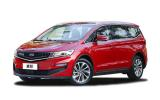• 指导价9.98-14.88万
• 长宽高4706×1909×1690
• 发动机1.5T|1.8T
• 变速箱6挡手动|6挡手自一体|7挡双离合
• 车质保4年或10万公里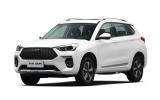• 指导价7.99-10.69万
• 长宽高4590*1845*1700
• 发动机1.5T
• 变速箱
• 车质保三年或10万公里• 指导价9.68-14.68万
• 长宽高4650*1850*1705
• 发动机1.5T
• 变速箱6档位手动|7挡双离合|6挡手自一体
• 车质保三年或10万公里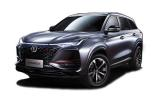• 指导价10.69-15.49万
• 长宽高4670*1865*1700
• 发动机1.5T|2.0T
• 变速箱6挡手动|6挡手自一体|8挡手自一体
• 车质保三年或10万公里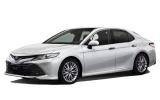• 指导价0.00起万
• 长宽高4885*1840*1455
• 发动机2.0L|2.5L
• 变速箱CVT无级变速(模拟10挡)
• 车质保三年或10万公里• 指导价7.39-10.50万
• 长宽高4410*1845*1695
• 发动机1.5T
• 变速箱6挡手动|7挡双离合
• 车质保三年或10万公里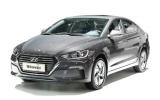• 指导价19.78-22.18万
• 长宽高4610*1800*1450
• 发动机1.6
• 变速箱6挡双离合
• 车质保三年或10万公里• 指导价8.98-15.18万
• 长宽高4545*1856*1668
• 发动机1.5T
• 变速箱手动(MT)|手自一体变速箱(AT）
• 车质保五年或15万公里• 指导价11.99-15.59万
• 长宽高4435*1850*1680
• 发动机2.0|1.4T
• 变速箱6挡手动|6挡手自一体
• 车质保五年或10万公里• 指导价10.88-12.58万
• 长宽高4660*1780*1450
• 发动机1.5L|1.4T
• 变速箱无级变速箱(CVT)
• 车质保三年或10万公里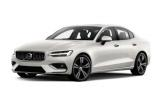• 指导价28.70-39.99万
• 长宽高4761*1850*1437
• 发动机2.0T
• 变速箱8挡手自一体
• 车质保三年不限公里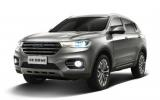• 指导价10.40-13.60万
• 长宽高4600*1860*1720
• 发动机1.5|2.0
• 变速箱7挡双离合
• 车质保三年或10万公里• 指导价34.98-39.98万
• 长宽高4900*1875*1900
• 发动机3.0
• 变速箱5挡手自一体
• 车质保五年或10万公里• 指导价8.48-11.28万
• 长宽高4419*1841*1616
• 发动机1.4T
• 变速箱5档手动|6档手自一体
• 车质保三年或10万公里

# 爱驰汽车焕新产品权益 24h全免费深度体验试到放心

4月1日,爱驰汽车官方推出系列直击人心的用户伙伴产品权益,针对近期购车、订车的用户提供包括置换补贴、半价充电、终身免流量费等十项新用户购车权益政策。

# 菲斯塔纯电动现在入手更划算

### 热车排行

• 全部
• 微型
• 小型
• 紧凑型
• 中型
• 中大型
• 豪华型
• SUV
• MPV
•# 东风悦达起亚河南普泽6S旗舰店

0371-86521188# 河南宇之林汽车销售服务有限公司

0371-55634444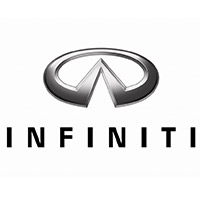# 河南威佳英菲尼迪4S店

0371—8888 0818# 郑州市金裕通汽车销售服务有限公司

0371—63832666# 河南锦堂盛沃尔沃4S中心

400-603-8683# 河南郑东天道长安福特4S旗舰店

0371-85333002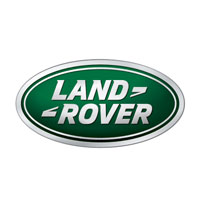# 河南通孚祥捷豹路虎4S中心

0371-66611888# 河南新裕隆东风悦达起亚4S店

0371-63563396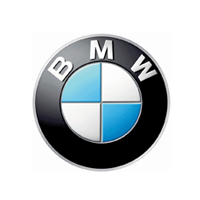# 郑州郑德宝汽车销售服务有限公司

4000663368-6268# 河南华星汽车贸易有限公司

0371-63788966

﻿
• 快速找车
• 选择品牌
• 选择品牌
• A  奥迪
• A  阿斯顿·马丁
• A  阿尔法·罗密欧
• B  宝沃
• B  布加迪
• B  巴博斯
• B  保时捷
• B  宾利
• B  奔驰
• B  宝马
• B  本田
• B  别克
• B  标致
• B  比亚迪
• B  宝骏
• B  北汽制造
• B  北汽新能源
• B  北汽幻速
• B  北汽威旺
• B  北京汽车
• B  奔腾
• B  北汽绅宝
• C  长安
• C  长安商用
• C  长城
• C  昌河
• D  大众
• D  道奇
• D  DS
• D  东南
• D  东风风神
• D  东风风行
• D  东风小康
• D  东风风度
• D  东风
• F  福特
• F  丰田
• F  菲亚特
• F  法拉利
• F  福田
• F  福迪
• F  福汽启腾
• G  观致
• G  广汽传祺
• G  广汽吉奥
• G  GMC
• H  红旗
• H  汉腾汽车
• H  哈弗
• H  哈飞
• H  海格
• H  海马
• H  华颂
• H  黄海
• H  华泰
• H  恒天
• J  吉利汽车
• J  捷豹
• J  Jeep
• J  江淮
• J  江铃
• J  金杯
• J  九龙
• J  金旅
• K  凯翼
• K  凯迪拉克
• K  克莱斯勒
• K  科尼塞克
• K  卡威
• K  开瑞
• L  路虎
• L  林肯
• L  劳斯莱斯
• L  兰博基尼
• L  雷克萨斯
• L  铃木
• L  雷诺
• L  理念
• L  力帆
• L  莲花汽车
• L  猎豹
• L  路特斯
• L  陆风
• M  马自达
• M  MG
• M  MINI
• M  玛莎拉蒂
• M  摩根
• M  迈凯轮
• N  纳智捷
• O  欧宝
• O  讴歌
• O  欧朗
• Q  奇瑞
• Q  起亚
• Q  启辰
• R  日产
• R  荣威
• R  瑞麒
• S  三菱
• S  斯威汽车
• S  萨博
• S  smart
• S  斯柯达
• S  斯巴鲁
• S  思铭
• S  双龙
• S  上汽大通
• S  双环
• T  特斯拉
• T  腾势
• W  沃尔沃
• W  五菱汽车
• W  五十铃
• W  威兹曼
• W  威麟
• X  现代
• X  雪佛兰
• X  雪铁龙
• X  西雅特
• Y  一汽
• Y  英菲尼迪
• Y  英致
• Y  依维柯
• Y  野马汽车
• Y  永源
• Z  众泰
• Z  中华
• Z  中兴
• Z  知豆
• 选择车系
• 选择车系
• 车型对比
• 选择品牌
• 选择品牌
• A  奥迪
• A  阿斯顿·马丁
• A  阿尔法·罗密欧
• B  宝沃
• B  布加迪
• B  巴博斯
• B  保时捷
• B  宾利
• B  奔驰
• B  宝马
• B  本田
• B  别克
• B  标致
• B  比亚迪
• B  宝骏
• B  北汽制造
• B  北汽新能源
• B  北汽幻速
• B  北汽威旺
• B  北京汽车
• B  奔腾
• B  北汽绅宝
• C  长安
• C  长安商用
• C  长城
• C  昌河
• D  大众
• D  道奇
• D  DS
• D  东南
• D  东风风神
• D  东风风行
• D  东风小康
• D  东风风度
• D  东风
• F  福特
• F  丰田
• F  菲亚特
• F  法拉利
• F  福田
• F  福迪
• F  福汽启腾
• G  观致
• G  广汽传祺
• G  广汽吉奥
• G  GMC
• H  红旗
• H  汉腾汽车
• H  哈弗
• H  哈飞
• H  海格
• H  海马
• H  华颂
• H  黄海
• H  华泰
• H  恒天
• J  吉利汽车
• J  捷豹
• J  Jeep
• J  江淮
• J  江铃
• J  金杯
• J  九龙
• J  金旅
• K  凯翼
• K  凯迪拉克
• K  克莱斯勒
• K  科尼塞克
• K  卡威
• K  开瑞
• L  路虎
• L  林肯
• L  劳斯莱斯
• L  兰博基尼
• L  雷克萨斯
• L  铃木
• L  雷诺
• L  理念
• L  力帆
• L  莲花汽车
• L  猎豹
• L  路特斯
• L  陆风
• M  马自达
• M  MG
• M  MINI
• M  玛莎拉蒂
• M  摩根
• M  迈凯轮
• N  纳智捷
• O  欧宝
• O  讴歌
• O  欧朗
• Q  奇瑞
• Q  起亚
• Q  启辰
• R  日产
• R  荣威
• R  瑞麒
• S  三菱
• S  斯威汽车
• S  萨博
• S  smart
• S  斯柯达
• S  斯巴鲁
• S  思铭
• S  双龙
• S  上汽大通
• S  双环
• T  特斯拉
• T  腾势
• W  沃尔沃
• W  五菱汽车
• W  五十铃
• W  威兹曼
• W  威麟
• X  现代
• X  雪佛兰
• X  雪铁龙
• X  西雅特
• Y  一汽
• Y  英菲尼迪
• Y  英致
• Y  依维柯
• Y  野马汽车
• Y  永源
• Z  众泰
• Z  中华
• Z  中兴
• Z  知豆
• 选择车系
• 选择车系
• 选择车型
• 选择车型
• 意见反馈